﻿ 简支梁横振动方程的稳定差分格式的构造 Construction of Stable Difference Schemes for Transverse Vibration Equations of Simply Supported Beam

Vol. 09  No. 01 ( 2020 ), Article ID: 33780 , 7 pages
10.12677/AAM.2020.91006

Construction of Stable Difference Schemes for Transverse Vibration Equations of Simply Supported Beam

Mingjun Li, Shaohong Du*

School of Mathematics and Statistics, Chongqing Jiaotong University, Chongqing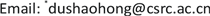Received: Dec. 12th, 2019; accepted: Dec. 28th, 2019; published: Jan. 3rd, 2020ABSTRACT

In this paper, we consider the construction of stable difference schemes for the transverse vibration equations for simply supported beam: Based on Taylor expansion, three-level difference explicit and implicit schemes are developed for fourth order problem, and the order of local truncation error for these two schemes is proven to be $O\left({\tau }^{2}+{h}^{2}\right)$. An artificial boundary condition is introduced in order to discrete the boundary condition that the perturbation vanishes at two ends of the simply supported beam. Owing to the discrete Fourier analysis, the explicit scheme is proven to be stable when the grid ratio $r=\frac{{a}^{2}{\tau }^{2}}{{h}^{4}}\le \frac{1}{8}$, and the implicit scheme is proven to be absolutely stable. Numerical experiments confirm the theoretical results.

Keywords:Transverse Vibration Equations for Simply Supported Beam, Finite Difference Method, Artificial Boundary Condition, Stability of Explicit and Implicit Schemes1. 引言

$\left\{\begin{array}{l}\frac{{\partial }^{2}u}{\partial {t}^{2}}+{a}^{2}\frac{{\partial }^{4}u}{\partial {x}^{4}}=0,00\\ {u|}_{t=0}=\phi \left(x\right),{\frac{\partial u}{\partial t}|}_{t=0}=\psi \left(x\right),0 (1.1)

2. 差分格式的构造

2.1. 三层差分显格式的构造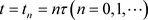生成矩形网格，网格节点为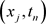，用表示定义在网点上的网函数。对(1.1)的第一个方程的左端在作Taylor展开有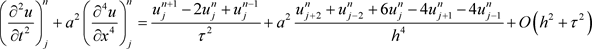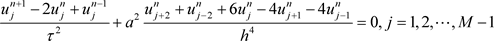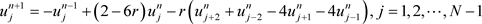。 (2.1)

2.2. 三层差分隐格式的构造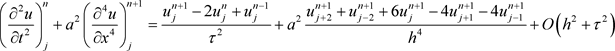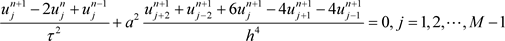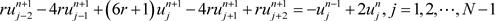。(2.2)

2.3. 人工边界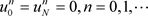。 (2.3)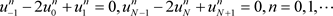。 (2.4)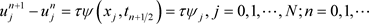。 (2.5)

3. 差分格式的稳定性分析

3.1. 显格式的稳定性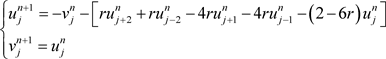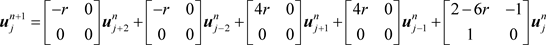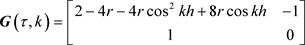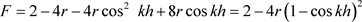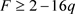。其特征方程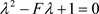的解为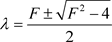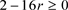时，增长矩阵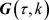的谱半径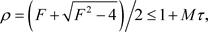其中，M为正常数。所以要使得对所有的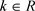，满足Von Neumann条件，即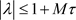，当且仅当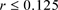3.2. 隐格式的稳定性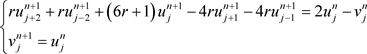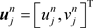，则上述方程组可写成：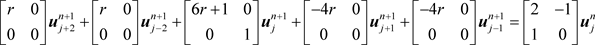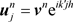，将其带入上述方程组并消去公因子，得到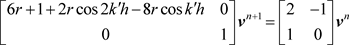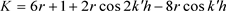，上述方程组化为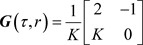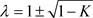4. 数值实验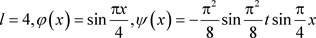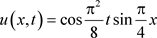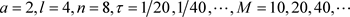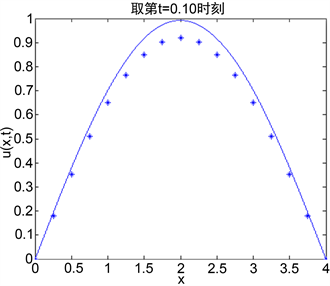Figure 1. Numerical solution obtained by the explicit scheme with different grid ratio, left: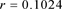，right:Table 1. Experiment convergence rate for the discrete maximum norm and -norm

5. 结论

Construction of Stable Difference Schemes for Transverse Vibration Equations of Simply Supported Beam[J]. 应用数学进展, 2020, 09(01): 43-49. https://doi.org/10.12677/AAM.2020.91006

1. 1. 查中伟. 数学物理偏微分方程[M]. 成都: 西南交通大学出版社, 2004.

2. 2. Du, S.H., Lin, R.C. and Zhang, Z.M. (2016) A Posteriori Error Analysis of Multipoint Flux Mixed Finite Element Methods for Interface Problems. Advances in Computational Mathematics, 42, 921-945.
https://doi.org/10.1007/s10444-015-9447-7

3. 3. Du, S.H., Sun, S.Y. and Xie, X.P. (2016) Residual-Based a Posteriori Error Estimation for Multipoint Flux Mixed Finite Element Methods. Numerische Mathematik, 134, 197-222.
https://doi.org/10.1007/s00211-015-0770-1

4. 4. Du, S.H. and Zhang, Z.M. (2015) A Robust Residual-Type a Posteriori Error Estimator for Convection-Diffusion Equations. Journal of Scientific Computing, 65, 138-170.
https://doi.org/10.1007/s10915-014-9972-4

5. 5. Du, S.H. and Xie, X.P. (2015) Convergence of Adaptive Mixed Finite Element Methods for Convection-Diffusion-Reaction Equations. Science China Mathematics, 58, 1327-1348.
https://doi.org/10.1007/s11425-015-4992-6

6. 6. Evans, D.J. and Alxiullah, A.R.B. (1983) Group Explicit Method for Parabolic Equations. International Journal of Computer Mathematics, 14, 73-105.
https://doi.org/10.1080/00207168308803377

7. 7. 崔嵬. 四阶抛物方程的一个区域分裂并行差分格式[J]. 科学技术与工程, 2008, 8(23): 6187-6190.

8. 8. 刘胜利, 张晔, 许世菊, 马富明. 四阶抛物方程的一个有限差分并行格式[J]. 吉林大学学报, 2005, 43(6): 725-731.

9. 9. 冯青华. 四阶抛物方程的一类交替分组方法[J]. 山东大学学报(自然科学版), 2007, 42(8): 79-82.

10. 10. 郭阁阳, 刘播. 四阶抛物方程一类变步长本性并行格式的稳定性分析[J]. 应用数学, 2008, 21(3): 485-489.

11. 11. 武文佳. 一类二维半线性椭圆边值问题的四阶紧有限差分格式[J]. 上海电机学院学报, 2013, 16(Z1): 88-93.

12. 12. 陈世平, 曾文平. 解四阶抛物型方程的三层高精度显格式[J]. 泉州师范学院学报, 2001, 19(6): 3-6.

13. 13. 陈世平. 解四阶抛物型方程的一族恒稳隐式格式[J]. 泉州师范学院学报,2002, 20(6):1-4.

14. 14. 曾文平. 四阶抛物型方程的三层恒稳差分格式[J]. 华侨大学学报(自然科学版), 2004, 25(4): 349-351.

15. 15. 邓世武, 贾雨, 姚兴苗. 基于四阶偏微分方程的光滑曲面重构方法[J]. 计算机应用, 2015, 35(2): 486-489.

16. NOTES

*通讯作者。